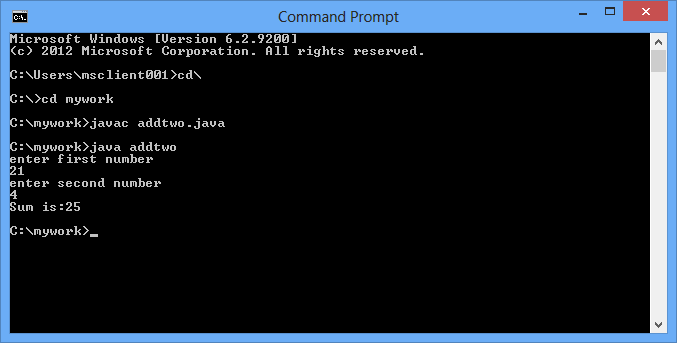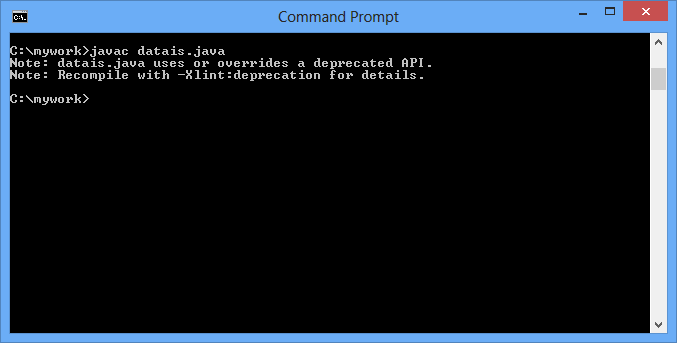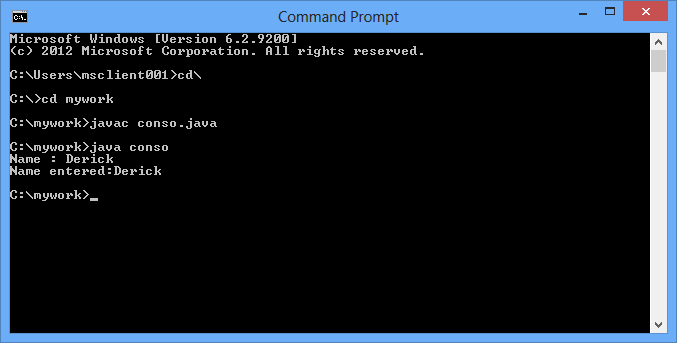The java platform provides various ways by which you can read an input. These are as follow:

1)      Using the Scanner class: We can take inputs from user using the scanner class as follow:

`import java.util.Scanner;class addscan{ public static void main(String arng[]){Scanner data = new Scanner(System.in);int num1, num2;System.out.println("Enter 1st number");num1 = data.nextInt();System.out.println("Enter 2nd number");num2 = data.nextInt();sum(num1,num2);}public static void sum(int numx, int numy){int sum=0;sum=numx+numy;System.out.println("Sum of 2 number:"+sum);}}`

This will produce the following output in command-Line:2)    Using the BufferedReader and InputStreamReader classes: We can take inputs from user using these class as follow:

`import java.io.*;class addtwo{public static void main(String args[]){BufferedReader br=new BufferedReader(new InputStreamReader(System.in));int x=0,y=0;try{System.out.println("enter first number");x=Integer.parseInt(br.readLine());System.out.println("enter second number");y=Integer.parseInt(br.readLine());}catch(IOException e){}System.out.println("Sum is:"+add(x,y));}public static int add(int x, int y){return (x+y);}}`

This will produce the following output in command-Line:3)      Using the DataInputStream classes: We can take inputs from user using these class as follow:

`import java.io.*;public class datais{  public static void main(String args[]) throws IOException  {    DataInputStream dis = new DataInputStream(System.in);    System.out.println("Enter your name: ");    String str1 = dis.readLine();    System.out.println("I know your name is " + str1);    dis.close();  }}`

This will produce the following output in command-Line:Since I have newer version of java, that’s why it is giving this note instead of the proper output. These APIs have been removed from the newer java because new classes have been added in place of it.

If you have an older version it will provide the following output:

Devesh

I know your name is Devesh

4)      Using the Console classes: We can take inputs from user using these class as follow:

`import java.io.Console;class conso{public static void main(String args[]){Console console = System.console();String s = console.readLine("Name : ");System.out.println("Name entered:" +s);}} `

This will produce the following output in command-Line:Modified On Mar-13-2018 06:06:17 AM